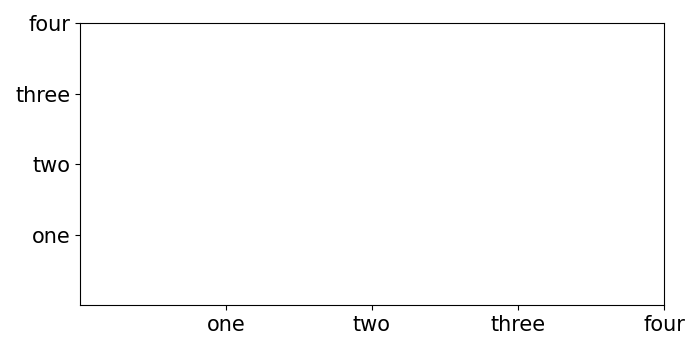# How to increase/reduce the fontsize of X and Y tick labels in Matplotlib?

MatplotlibWX PythonData Visualization

#### Python Data Science basics with Numpy, Pandas and Matplotlib

Most Popular

63 Lectures 6 hours

#### Data Visualization using MatPlotLib & Seaborn

11 Lectures 4 hours

#### MatPlotLib with Python

9 Lectures 2.5 hours

To increase/reduce the fontsize of x and y tick labels in matplotlib, we can initialize the fontsize variable to reduce or increase font size.

## Steps

• Create a list of numbers (x) that can be used to tick the axes.

• Get the axis using subplot() that helps to add a subplot to the current figure.

• Set ticks on x and y axes using set_xticks and set_yticks methods respectively and list x (from step 1).

• Set tick labels with label lists (["one", "two", "three", "four"]) using set_xticklabels() and set_yticklabels() with fontsize variable.

• To add space between axes and tick labels, we can use tick_params() method with pad argument that helps to add space. Argument direction (in) helps to put ticks inside the axes, and axis (both), parameters to be applied on both the axes.

• To show the figure, use plt.show() method.

## Example

from matplotlib import pyplot as plt
plt.rcParams["figure.figsize"] = [7.00, 3.50]
plt.rcParams["figure.autolayout"] = True
x = [1, 2, 3, 4]
ax1 = plt.subplot()
ax1.set_xticks(x)
ax1.set_yticks(x)
fontsize = 15
ax1.set_xticklabels(["one", "two", "three", "four"], fontsize=fontsize)
ax1.set_yticklabels(["one", "two", "three", "four"], fontsize=fontsize)
plt.show()

## Output